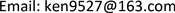1. 引言

2. 理论模型

W M = μ I ⋅ B (1)

WM可取值为所有氢原子能级精细结构 Δ E 中值最小的  。因此，核外电子自旋与轨道运动在质子处产生的磁场为

B = Δ E μ I (2)

H = q m 4 π μ 0 r 2 (3)

r可取为波尔半径，即 r = 0.529 × 10 − 10 m ，该场可视为核外电子磁场在质子上的感应场，即

H = B μ 0 (4)

Δ E μ I μ 0 = q m 4 π r 2 μ 0 (5)

q m = 4 π r 2 Δ E μ I (6)

F M = q m 2 4 π μ 0 r 2 (7)

F E = q 2 4 π ε 0 r 2 (8)

μ 0 , ε 0 为真空磁导率和介电常数。因此可导出自旋磁力与库仑力之比为

| F M | | F E | = ε 0 μ 0 ⋅ q m 2 q 2 (9)

S运动后也击中d点质子，即在磁引力作用下反应概率会有所提高，Ra可定义为全极化条件下自旋引力的作用半径。令 δ = | F M | | F E | − 1 ，δ为磁作用力超出电作用力的比例系数。当圆环上入射的质子能量为E0时，其x向位移与y向位移大致有如下关系

x = V 0 2 y m p F δ (10)

F δ = δ F M = δ q m 2 4 π μ 0 [ ( L − x ) 2 + y 2 ] (11)

L 2 = R a 3 8 π μ 0 m p V 0 2 δ q m 2 (12)

R a = δ L 2 q m 2 8 π μ 0 m p v 0 2 3 = δ L 2 q m 2 8 π μ 0 E 0 3 (13)

ζ = π R a r (14)

P = 1 − 1 e σ N x (15)

ζ = P 2 / P 1 = e σ 1 N x ( e σ 2 N x − 1 ) e σ 2 N x ( e σ 1 N x − 1 ) (16)

Δ σ = σ 2 − σ 1 = − ln [ e σ 1 N x − ( e σ 1 N x − 1 ) ζ ] N x (17)

3. 算例3.1. 模型校验

3.2. 自旋引起的反应截面增量分析

3.3. 自旋对低能区反应概率的影响

4. 结束语